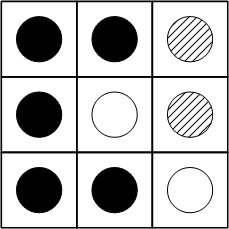## Ken's Puzzle of the WeekPatterns of Circles in a GridFill a grid with black, white and gray circles, such that: Each black circle neighbors an even number of black circles (0 may be considered even.) Each white circle neighbors an odd number of white circles. Each gray circle neighbors an equal number of black and white circles. An example 3x3 grid is shown.

Try to solve the puzzle for 3x3 to 6x6 grids with the following different requirements:

1. Maximize the number of black circles.
2. Maximize the number of white circles.
3. Minimize the number of gray circles. Can a grid be made without any?
4. Evenly distribute the circles. That is, minimize the difference between the max and min colors.

Source: Original.
Solution
Mail to Ken